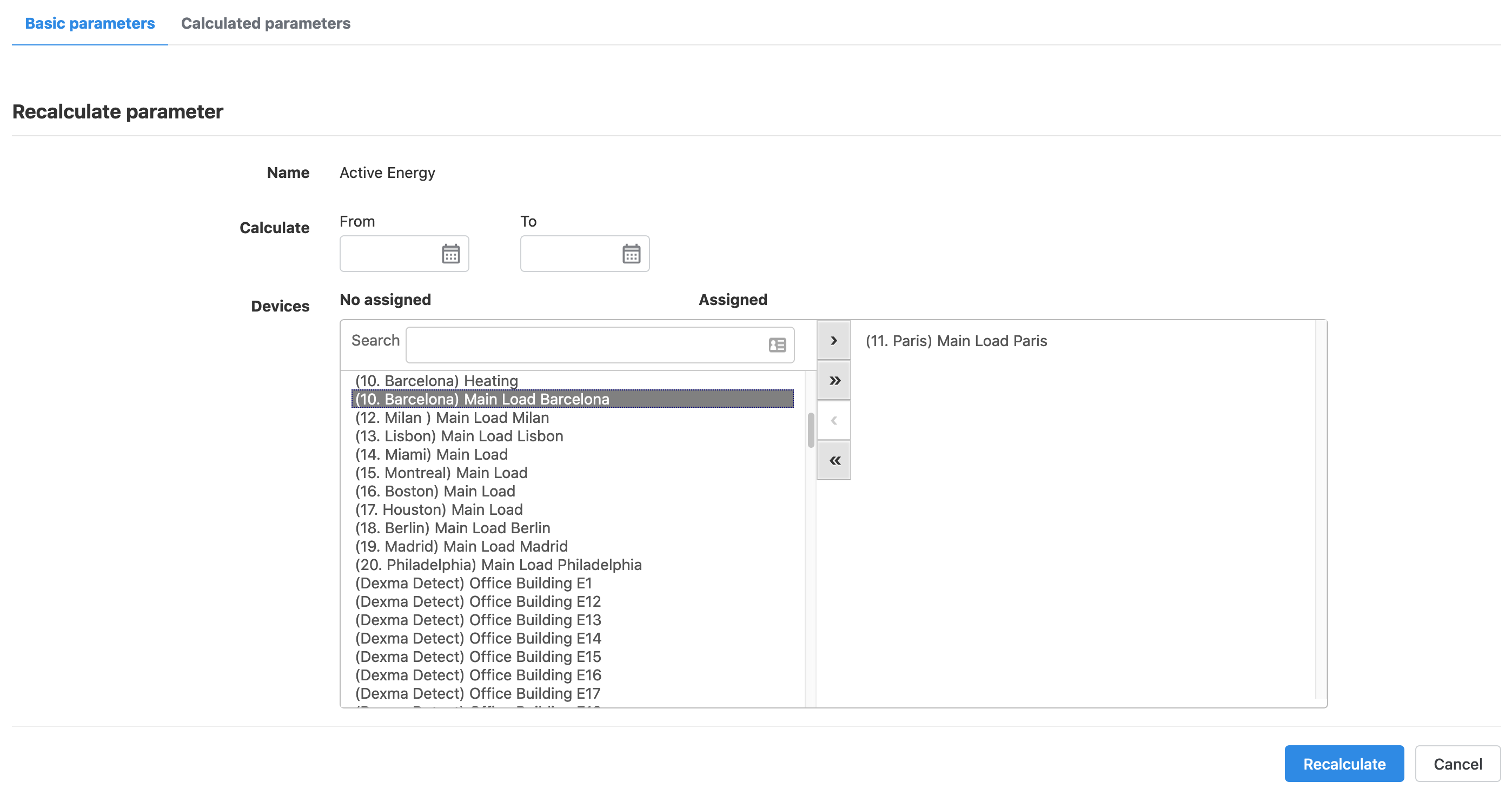# Recalculate parameters

## Introduction

In this article you are going to learn how to recalculate a parameter in the Platform.

## Requirements

For a parameter to be available to recalculate, it must previously exist in the account. For a parameter to be available you need to insert data for that parameter in a device.

## How does it work?

In order to recalculate a parameter, go to Configuration -> Parameters.There are 2 tabs in this menu, Basic parameters and Calculated parameters. Recalculation is performed in the Basic parameters tab.

Click Recalculate on the parameter that you want to recalculate. The following menu will appear:Select the start and end date for the period where you want to recalculate the parameters, and select which parameters from the left list. Assign/unassign them for recalculation using the arrows in the middle. Once the list on your right contains all the parameters to recalculate, hit the Recalculate button. This will launch the recalculation process, that can last several minutes, depending on the amount of data and the frequencies to recalculate.

### Notes

• The recalculation period is limited to one year. If you need to recalculate data for periods over a year, you will have to launch a recalculation process for each year of data.
• Recalculation for the devices created via Virtual Devices app is different and should be performed directly in the app.

## Tips and tricks

Use the recalculation feature in the following cases:

• If you have historic temperature data and you want to calculate degree days data for an existing degree days device.
• If you have peaks in your data, and have introduced new data to remove the peak, you might need to run the recalculation process
• If you have a large data gap between 2 readings, and you inserted a new reading in between them